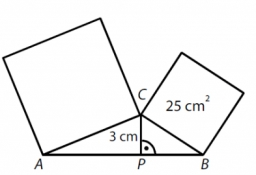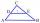# Squares above sides

Two squares are constructed on two sides of the ABC triangle. The square area above the BC side is 25 cm2. The height vc to the side AB is 3 cm long. The heel P of height vc divides the AB side in a 2: 1 ratio. The AC side is longer than the BC side. Calculate the length of the AB side in cm.
Calculate the area of the square above the AC side in cm2.

c =  12 cm
S2 =  73 cm2

### Step-by-step explanation:

Try calculation via our triangle calculator.Did you find an error or inaccuracy? Feel free to write us. Thank you!Tips to related online calculators
Pythagorean theorem is the base for the right triangle calculator.

#### You need to know the following knowledge to solve this word math problem:

We encourage you to watch this tutorial video on this math problem:

## Related math problems and questions:

• RhumblineFind circumference and area of the rhumbline ABCD if the short side AD of which has a length of 5 cm, and the heel of the height from D leading to the AB side divides the AB side into two sections of 3 cm and 4 cm.
• Side lengthsIn the triangle ABC, the height to the side a is 6cm. The height to side b is equal to 9 cm. Side "a" is 4 cm longer than side "b". Calculate the side lengths a, b.
• CalculateCalculate the height to the base of the isosceles triangle ABC if the length of the base is c = 24cm and the arms have a length b = 13cm.
• Isosceles triangle 9Given an isosceles triangle ABC where AB= AC. The perimeter is 64cm, and the altitude is 24cm. Find the area of the isosceles triangle.
• Rectangular triangle PQRIn the rectangular triangle PQR, the PQ leg is divided by the X point into two segments of which longer is 25cm long. The second leg PR has a length 16 cm. The length of the RX is 20 cm. Calculate the length p of side RQ. The result is round to 2 decimal
• Recursion squaresIn the square, ABCD has inscribed a square so that its vertices lie at the centers of the sides of the square ABCD. The procedure of inscribing the square is repeated this way. The side length of the square ABCD is a = 22 cm. Calculate: a) the sum of peri
• Squares ratioThe first square has a side length of a = 6 cm. The second square has a circumference of 6 dm. Calculate the proportions of the perimeters and the proportions of the contents of these squares? (Write the ratio in the basic form). (Perimeter = 4 * a, conte
• Equilateral triangleThe equilateral triangle has a 23 cm long side. Calculate its content area.
• Right ΔA right triangle has the length of one leg 11 cm and the hypotenuse 61 cm size. Calculate the height of the triangle.
• Rectangle 3-4-5The sides of the rectangle are in a ratio of 3:4. The length of the rectangle diagonal is 20 cm. Calculate the content of the rectangle.
• The right triangleThe right triangle ABC has a leg a = 36 cm and an area S = 540 cm2. Calculate the length of the leg b and the median t2 to side b.
• Segment in a triangleIn a triangle ABC with the side/AB/ = 24 cm is constructed middle segment/DE/ = 18 cm parallel to the side AB at a distance 1 cm from AB. Calculate the height of the triangle ABC to side AB.
• Sum of squaresThe sum of squares above the sides of the rectangular triangle is 900 cm2. Calculate content of square over the triangle's hypotenuse.
• CalculateCalculate the cone's surface and volume that results from the rotation of the right triangle ABC with the squares 6 cm and 9 cm long around the shorter squeegee.
• In aIn a right triangle, the areas of the squares above its sides are 169; 25 and 144. The length of its longer leg is:
• Right isoscelesCalculate area of the isosceles right triangle which perimeter is 26 cm.
• AP RT triangleThe length of the sides of a right triangle forms an arithmetic progression, and the longer leg is 24 cm long. What are the perimeter and area?